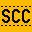# scc

simple c99 compiler
git clone git://git.simple-cc.org/scc

unpack.c (2968B)

```      1 #include <ctype.h>
2 #include <stdarg.h>
3 #include <stdio.h>
4 #include <stdlib.h>
5
6 #include <scc/mach.h>
7 #include "libmach.h"
8
9 static int
10 lunpack(unsigned char *src, char *fmt, va_list va)
11 {
12 	unsigned char *bp, *cp;
13 	unsigned short *sp;
14 	unsigned s;
15 	unsigned long *lp, l;
16 	unsigned long long *qp, q;
17 	int n;
18
19 	bp = src;
20 	while (*fmt) {
21 		switch (*fmt++) {
22 		case '\'':
23 			n = atoi(fmt);
24 			while (isdigit(*fmt))
25 				fmt++;
26 			cp = va_arg(va, unsigned char *);
27 			while (n--)
28 				*cp++ = *bp++;
29 			break;
30 		case 'c':
31 			cp = va_arg(va, unsigned char *);
32 			*cp = *bp++;
33 			break;
34 		case 's':
35 			sp = va_arg(va, unsigned short *);
36 			s =  (unsigned) *bp++;
37 			s |= (unsigned) *bp++ << 8;
38 			*sp = s;
39 			break;
40 		case 'l':
41 			lp = va_arg(va, unsigned long *);
42 			l = (unsigned long) *bp++;
43 			l |= (unsigned long) *bp++ << 8;
44 			l |= (unsigned long) *bp++ << 16;
45 			l |= (unsigned long) *bp++ << 24;
46 			*lp = l;
47 			break;
48 		case 'q':
49 			qp = va_arg(va, unsigned long long *);
50 			q = (unsigned long long) *bp++;
51 			q |= (unsigned long long) *bp++ << 8;
52 			q |= (unsigned long long) *bp++ << 16;
53 			q |= (unsigned long long) *bp++ << 24;
54 			q |= (unsigned long long) *bp++ << 32;
55 			q |= (unsigned long long) *bp++ << 40;
56 			q |= (unsigned long long) *bp++ << 48;
57 			q |= (unsigned long long) *bp++ << 56;
58 			*qp = q;
59 			break;
60 		default:
61 			va_end(va);
62 			return -1;
63 		}
64 	}
65
66 	return bp - src;
67 }
68
69 static int
70 bunpack(unsigned char *src, char *fmt, va_list va)
71 {
72 	unsigned char *bp, *cp;
73 	unsigned short *sp;
74 	unsigned s;
75 	unsigned long *lp, l;
76 	unsigned long long *qp, q;
77 	int n;
78
79 	bp = src;
80 	while (*fmt) {
81 		switch (*fmt++) {
82 		case '\'':
83 			n = atoi(fmt);
84 			while (isdigit(*fmt))
85 				fmt++;
86 			cp = va_arg(va, unsigned char *);
87 			while (n--)
88 				*cp++ = *bp++;
89 			break;
90 		case 'c':
91 			cp = va_arg(va, unsigned char *);
92 			*cp = *bp++;
93 			break;
94 		case 's':
95 			sp = va_arg(va, unsigned short *);
96 			s =  (unsigned) *bp++ << 8;
97 			s |= (unsigned) *bp++;
98 			*sp = s;
99 			break;
100 		case 'l':
101 			lp = va_arg(va, unsigned long *);
102 			l =  (unsigned long) *bp++ << 24;
103 			l |= (unsigned long) *bp++ << 16;
104 			l |= (unsigned long) *bp++ << 8;
105 			l |= (unsigned long) *bp++;
106 			*lp = l;
107 			break;
108 		case 'q':
109 			qp = va_arg(va, unsigned long long *);
110 			q =  (unsigned long long) *bp++ << 56;
111 			q |= (unsigned long long) *bp++ << 48;
112 			q |= (unsigned long long) *bp++ << 40;
113 			q |= (unsigned long long) *bp++ << 32;
114 			q |= (unsigned long long) *bp++ << 24;
115 			q |= (unsigned long long) *bp++ << 16;
116 			q |= (unsigned long long) *bp++ << 8;
117 			q |= (unsigned long long) *bp++;
118 			*qp = q;
119 			break;
120 		default:
121 			va_end(va);
122 			return -1;
123 		}
124 	}
125
126 	return bp - src;
127 }
128
129 int
130 unpack(int order, unsigned char *src, char *fmt, ...)
131 {
132 	int r;
133         int (*fn)(unsigned char *dst, char *fmt, va_list va);
134         va_list va;
135
136         va_start(va, fmt);
137         fn = (order == LITTLE_ENDIAN) ? lunpack : bunpack;
138         r = (*fn)(src, fmt, va);
139         va_end(va);
140
141         return r;
142 }
```